# RD Sharma Solutions for Class 11 Maths Chapter 11 Trigonometric Equations

## RD Sharma Solutions Class 11 Maths Chapter 11 – Get Free PDF (for 2021-22)

RD Sharma Solutions for Class 11 Maths Chapter 11 – Trigonometric Equations is given here for students to score good marks in the board exams. This chapter gives an overview of Trigonometric Equations (the equations containing trigonometric functions of unknown angles are known as trigonometric equations). For students who face difficulty in understanding the concepts during class hours, the experts at BYJU’S have developed the solutions based on the students’ grasping abilities. RD Sharma Solutions strictly follows the latest CBSE syllabus directed by the CBSE board.

Students can solve both chapter-wise and exercise-wise problems to increase their confidence level before appearing for the board exam. To boost interest among students in this chapter, RD Sharma Solutions for Class 11 Chapter 11 Trigonometric Equations pdf links are given here for easy access.

Chapter 11 – Trigonometric Equations contain one exercise and the RD Sharma Solutions present in this page provide solutions to the questions present in this exercise. Now, let us have a look at the concepts discussed in this chapter.

• Some definitions.
• General solutions of trigonometric equations.
• General solutions of trigonometric equations in specific forms.

## Download the Pdf of RD Sharma Solutions for Class 11 Maths Chapter 11 – Trigonometric Equations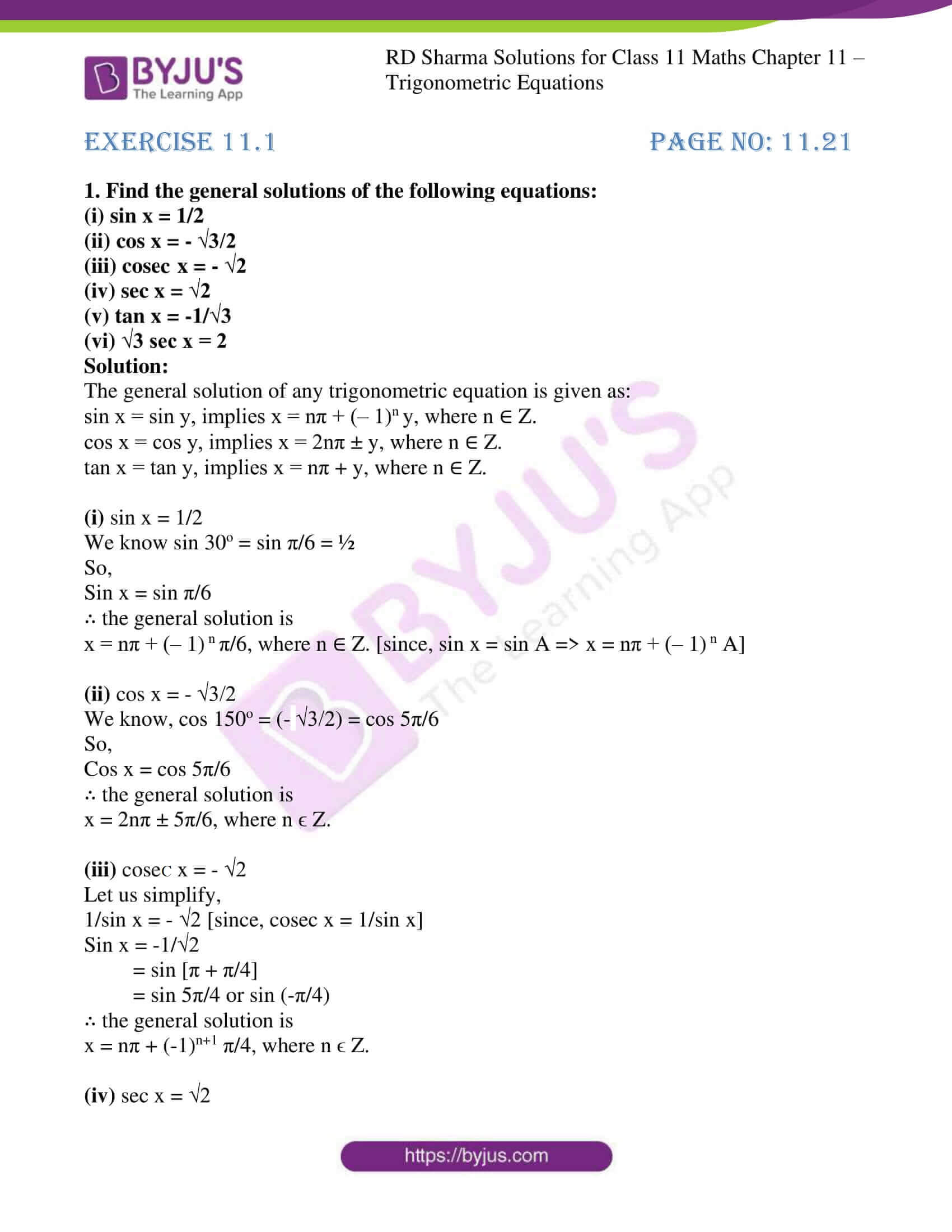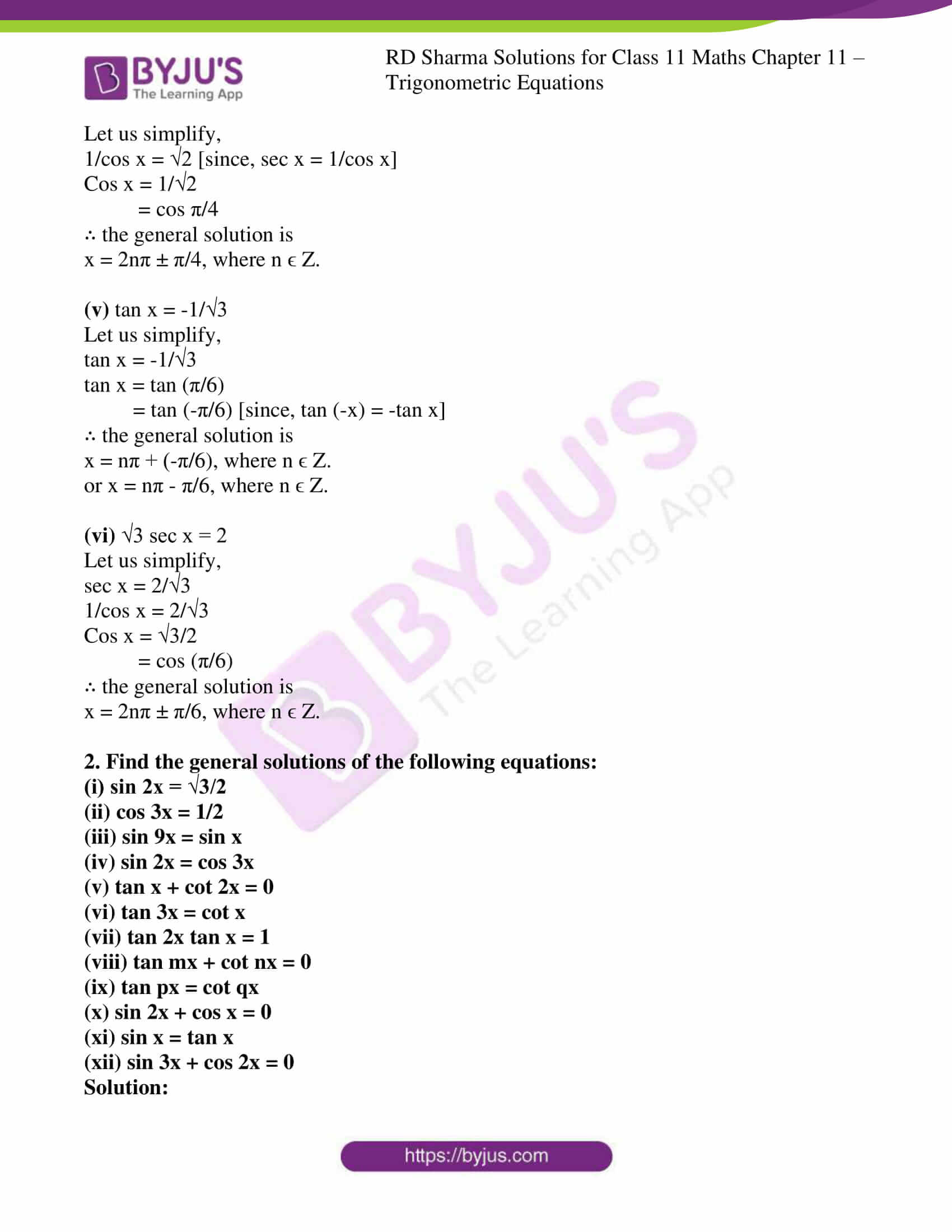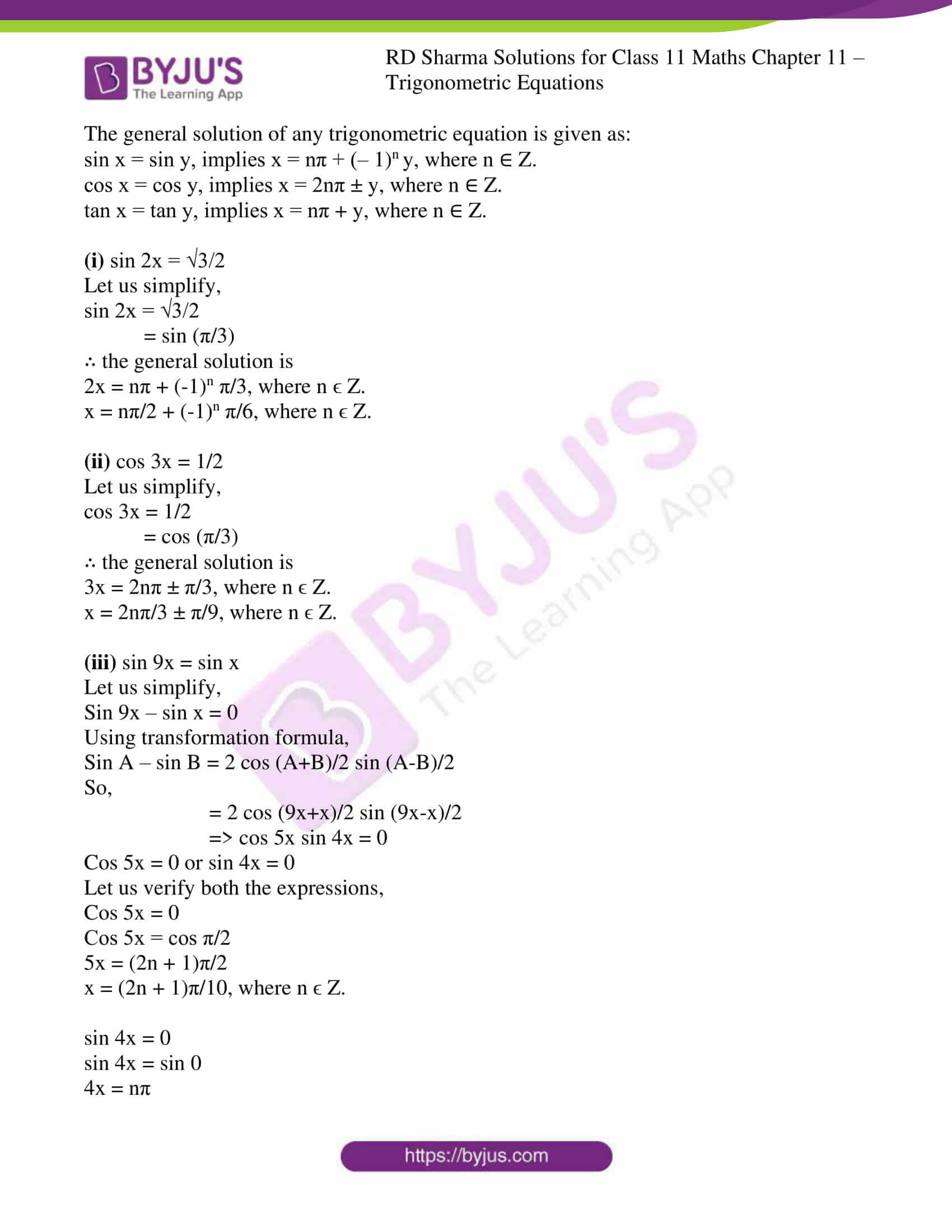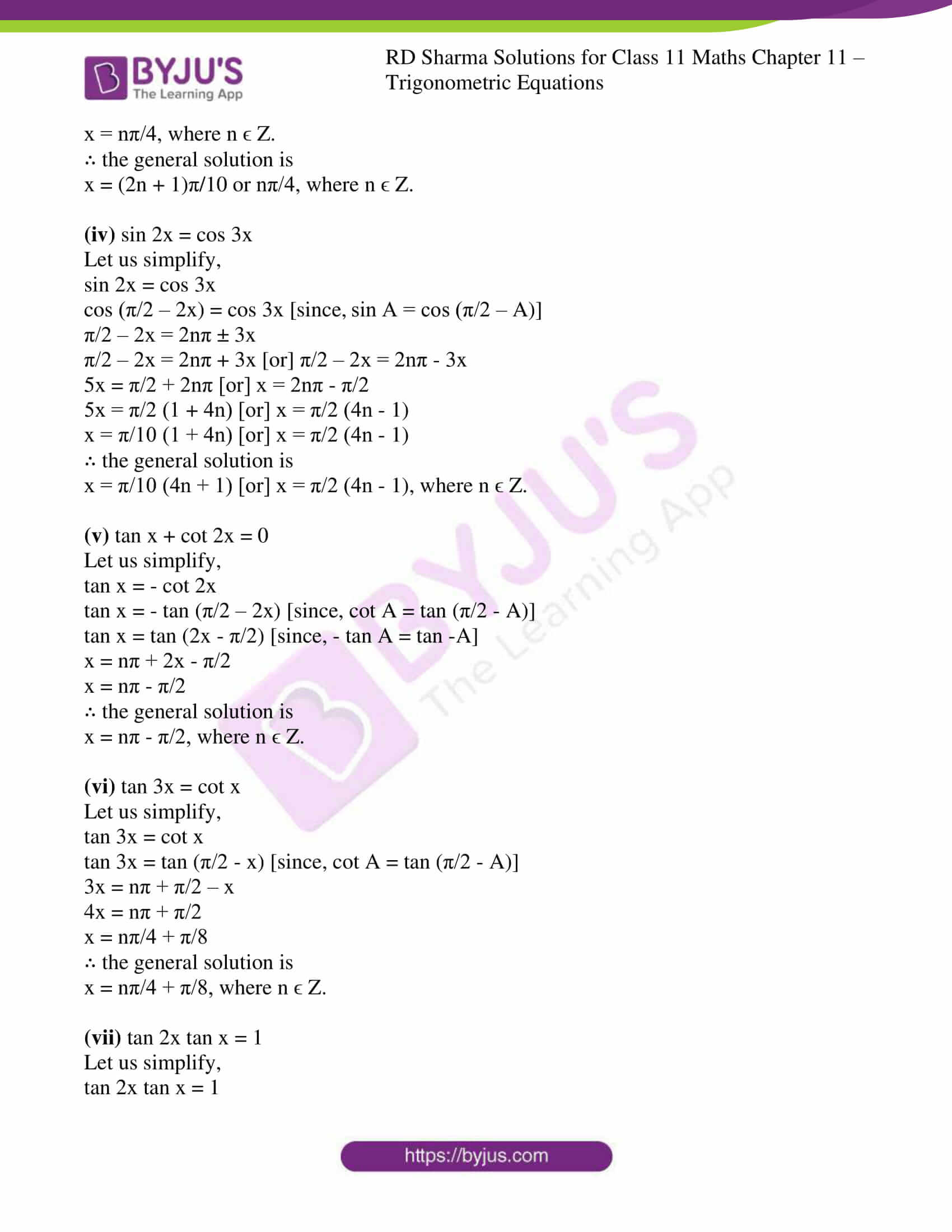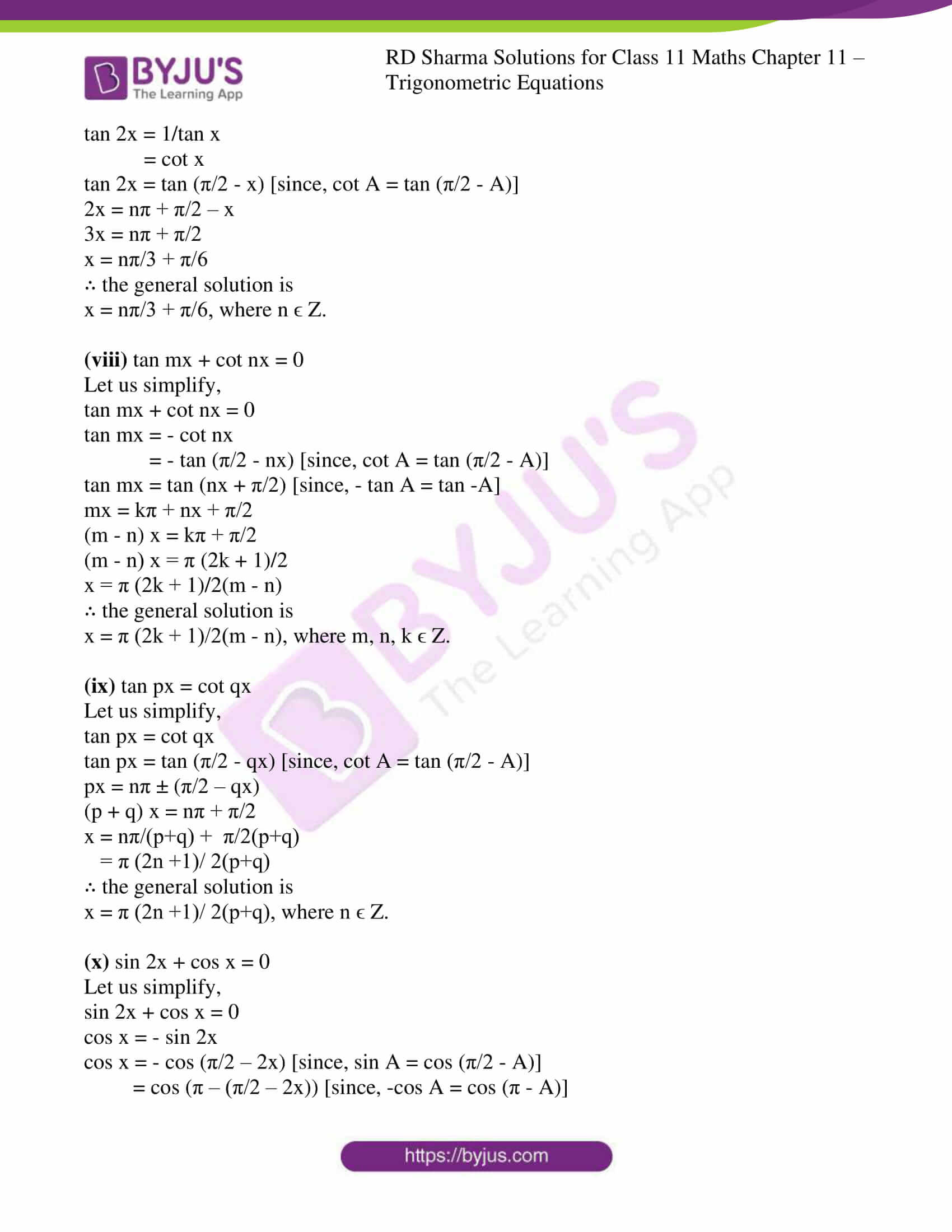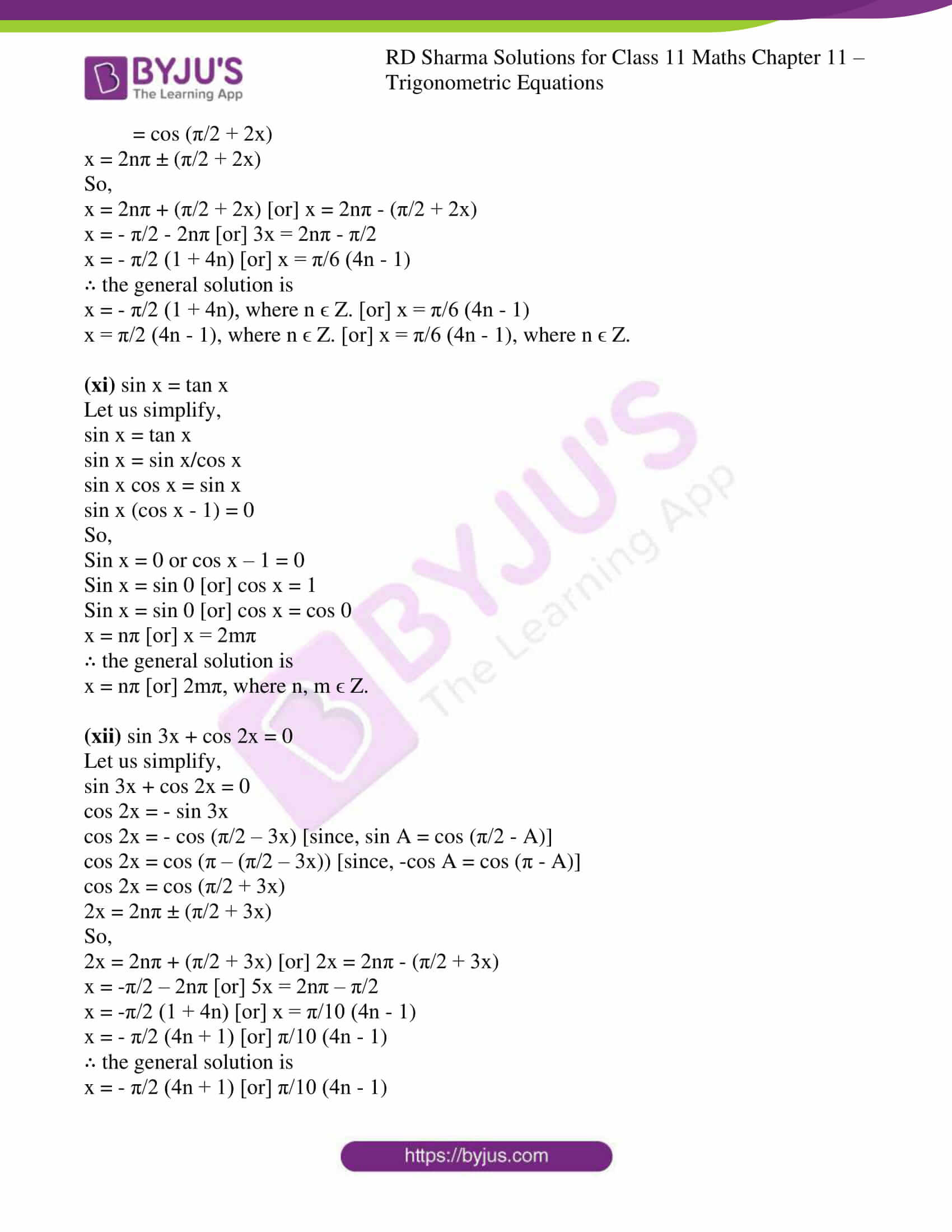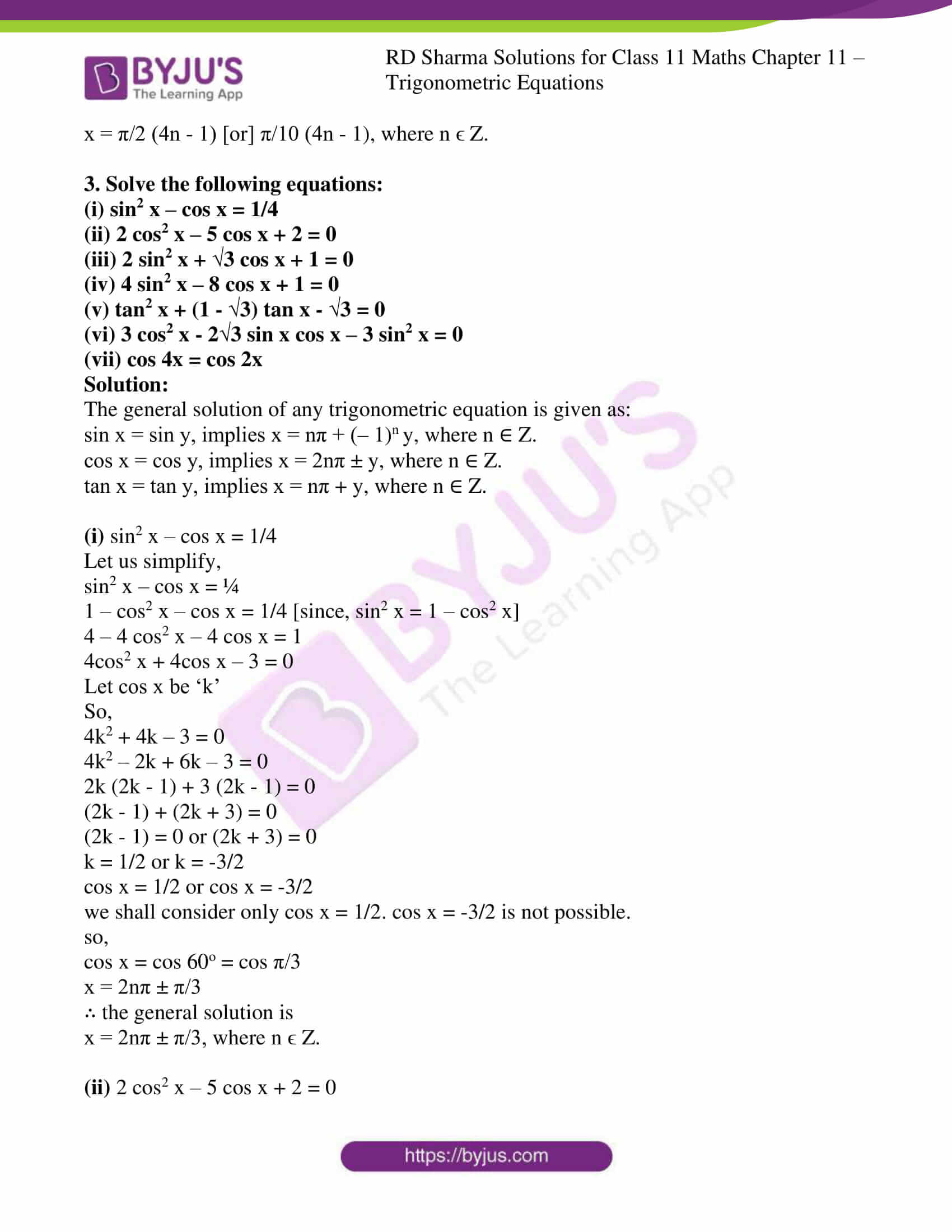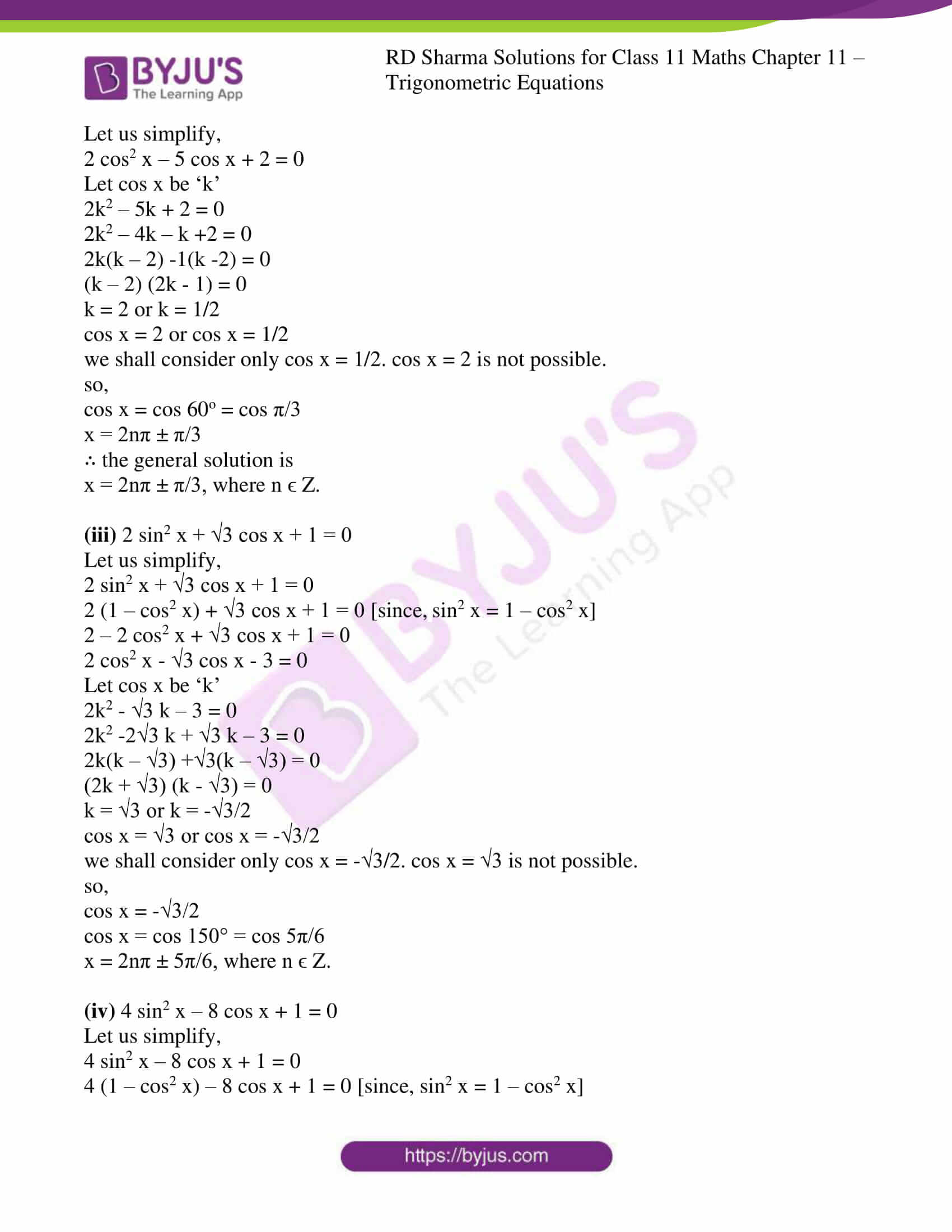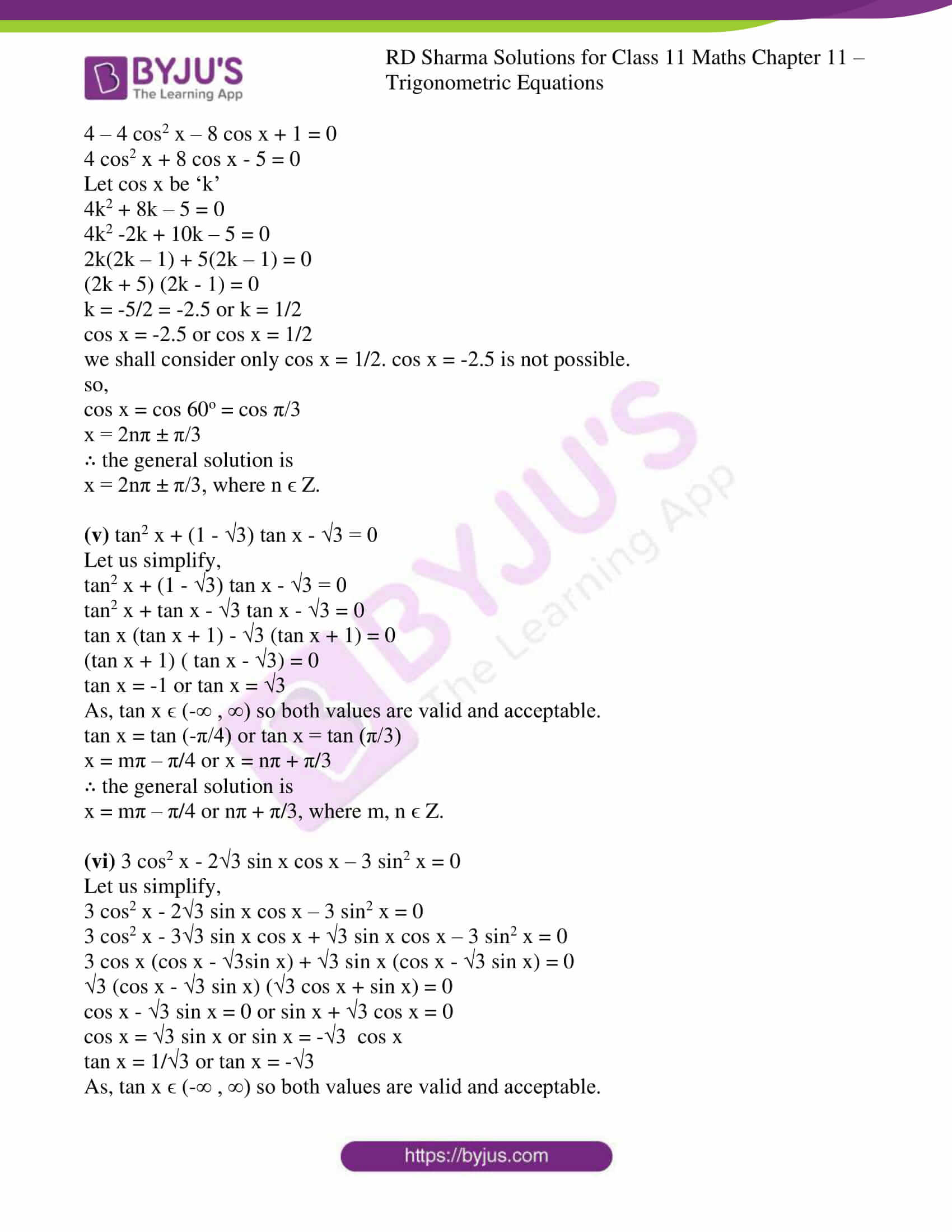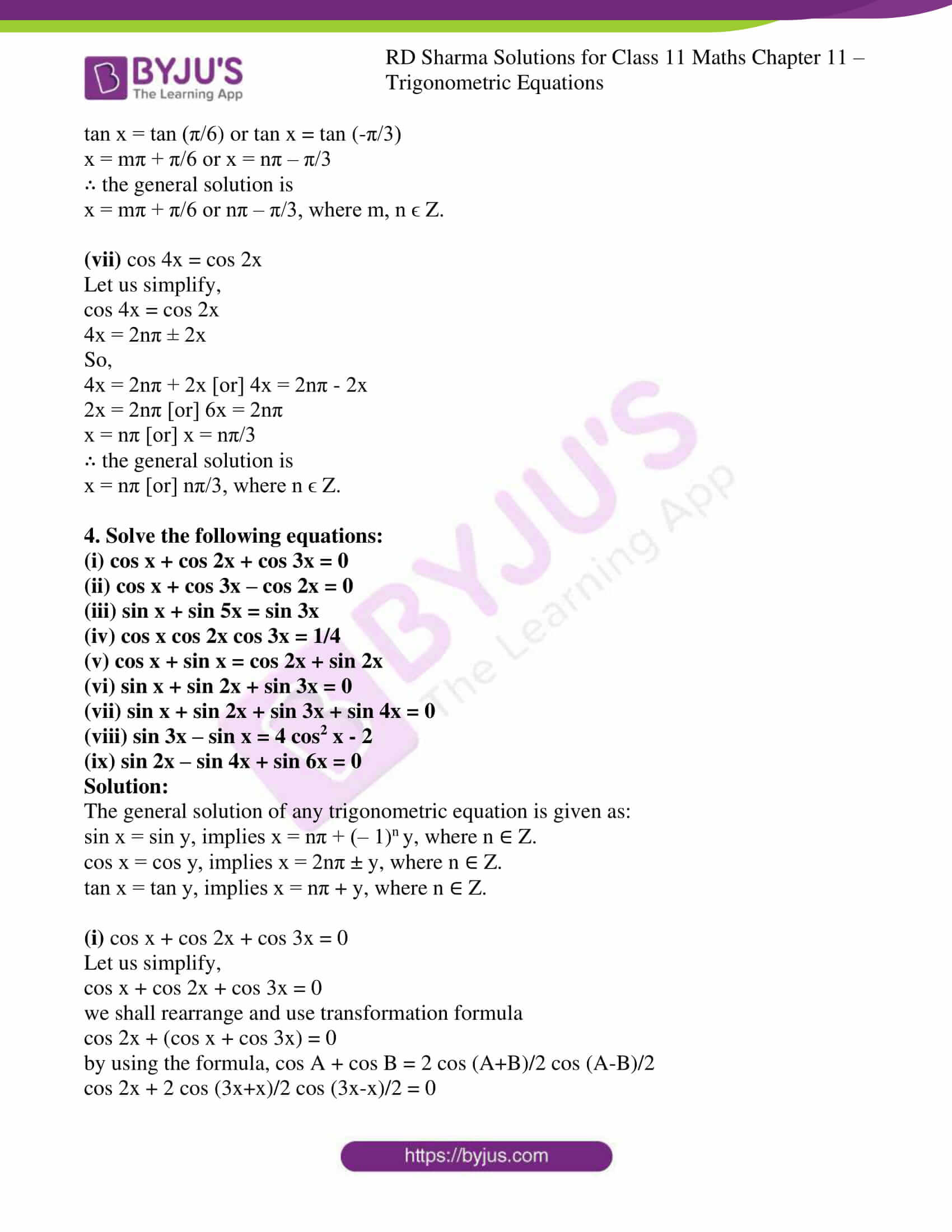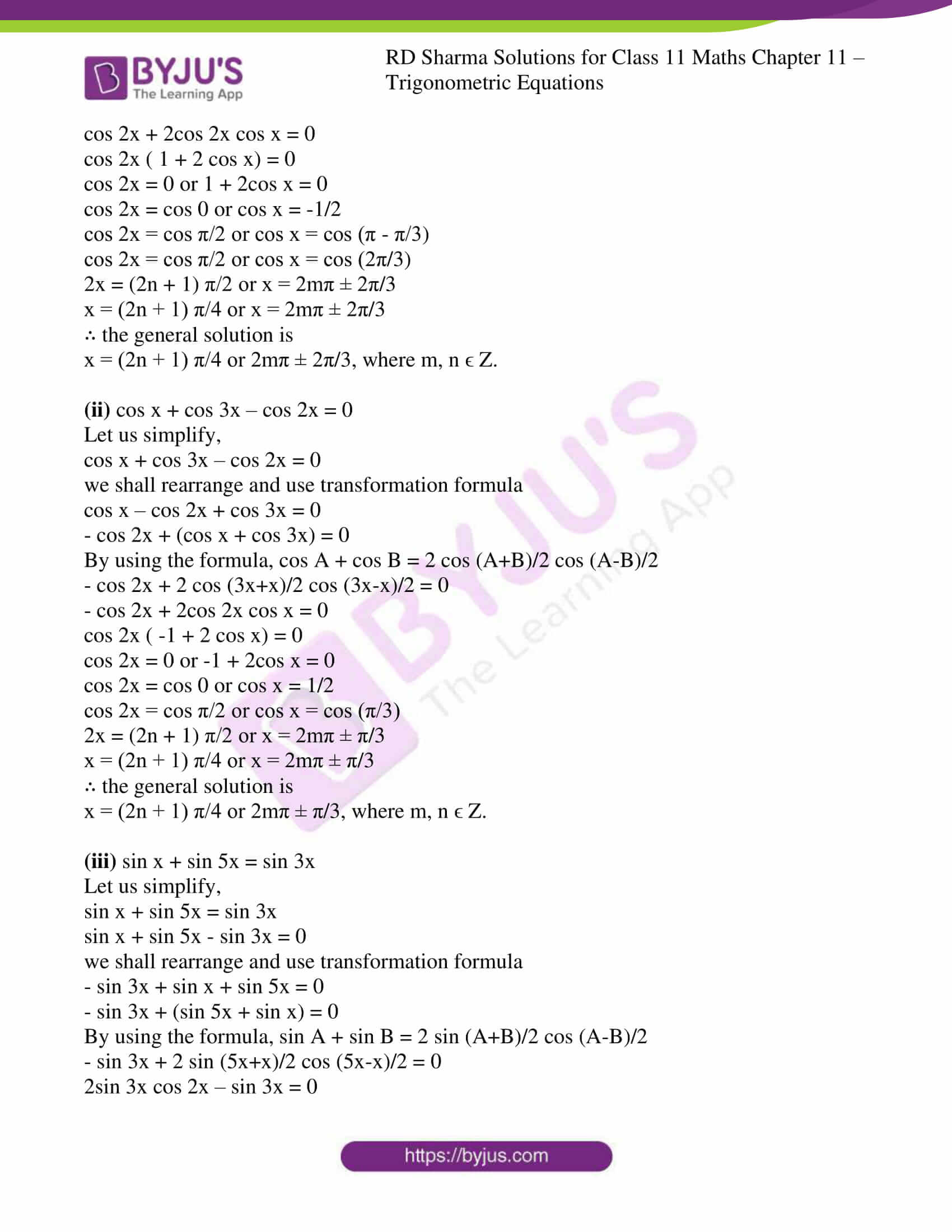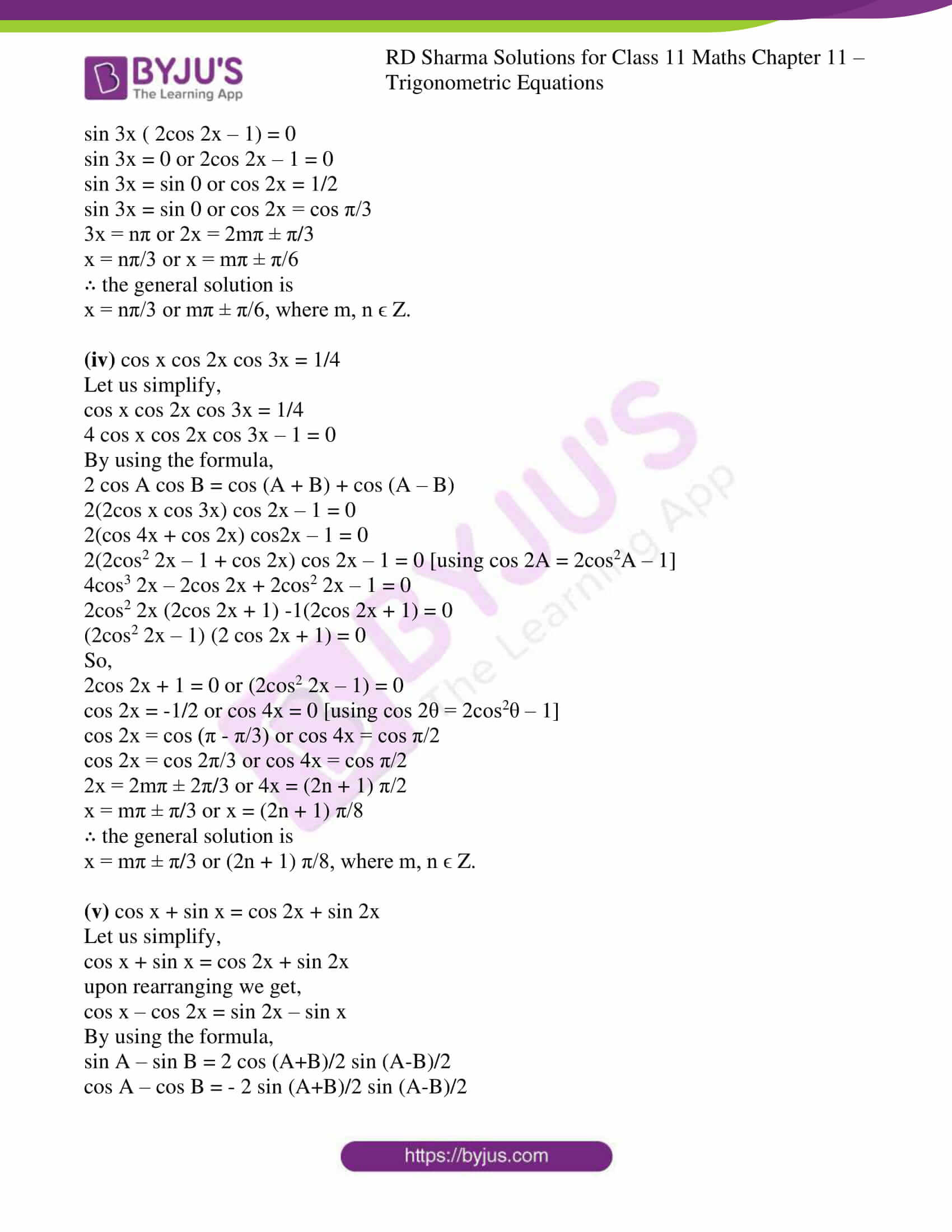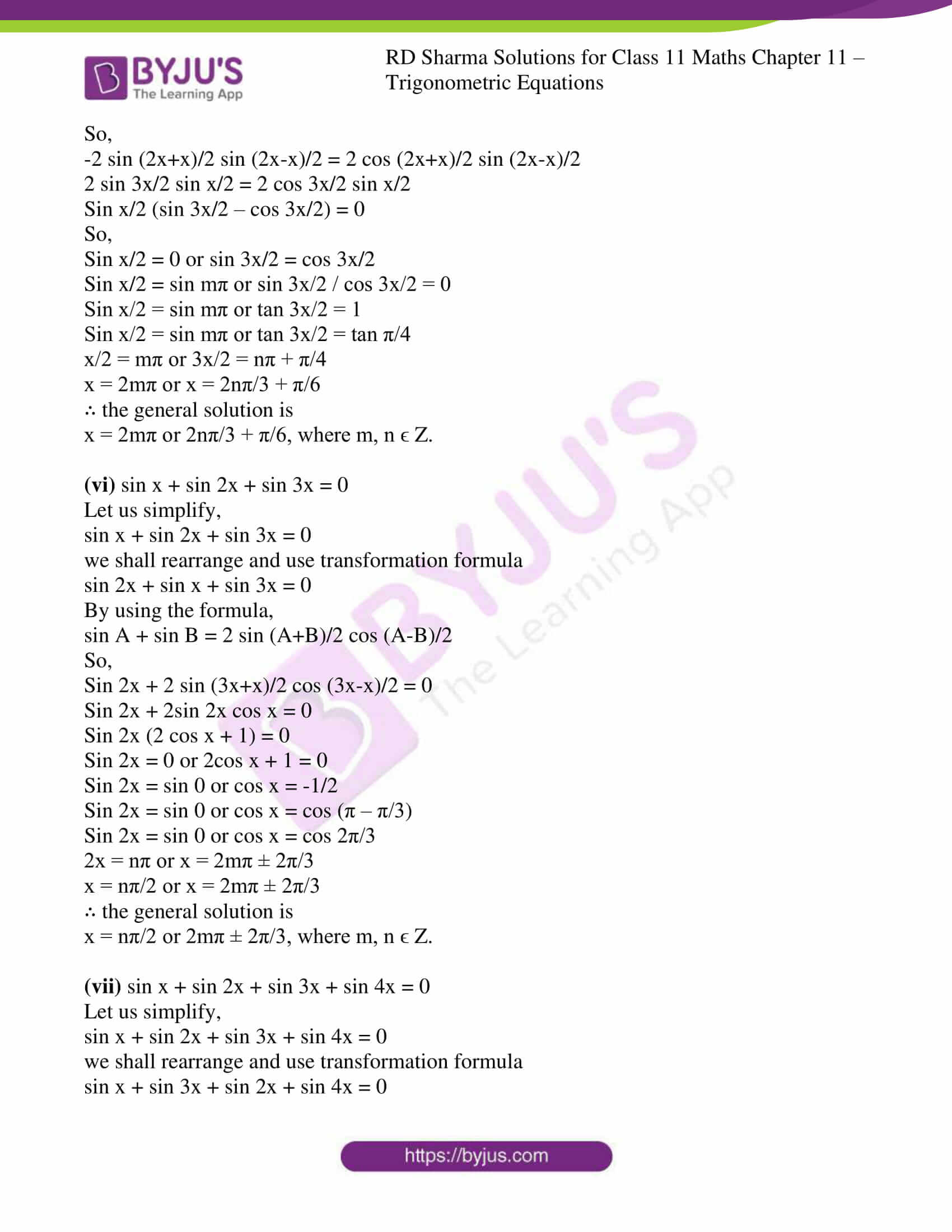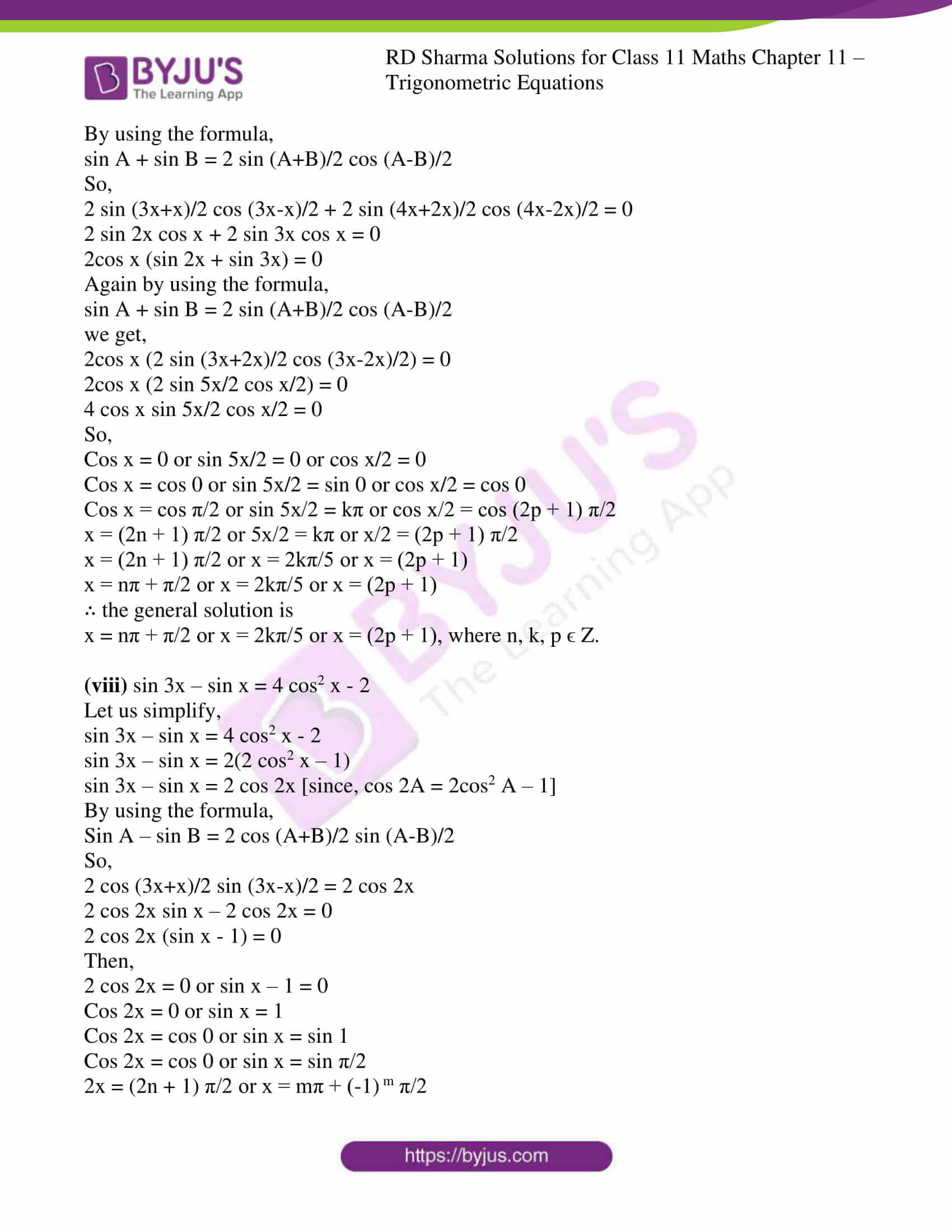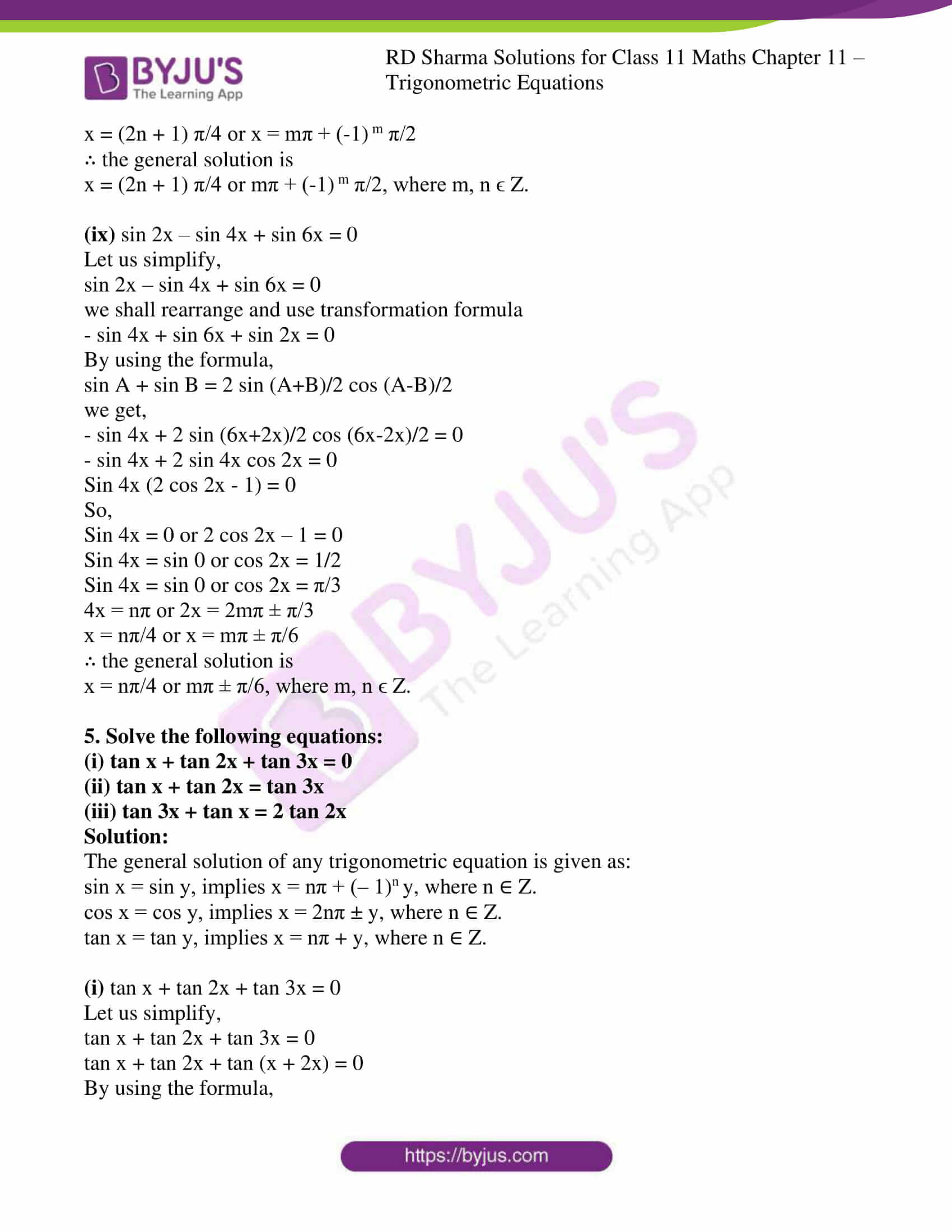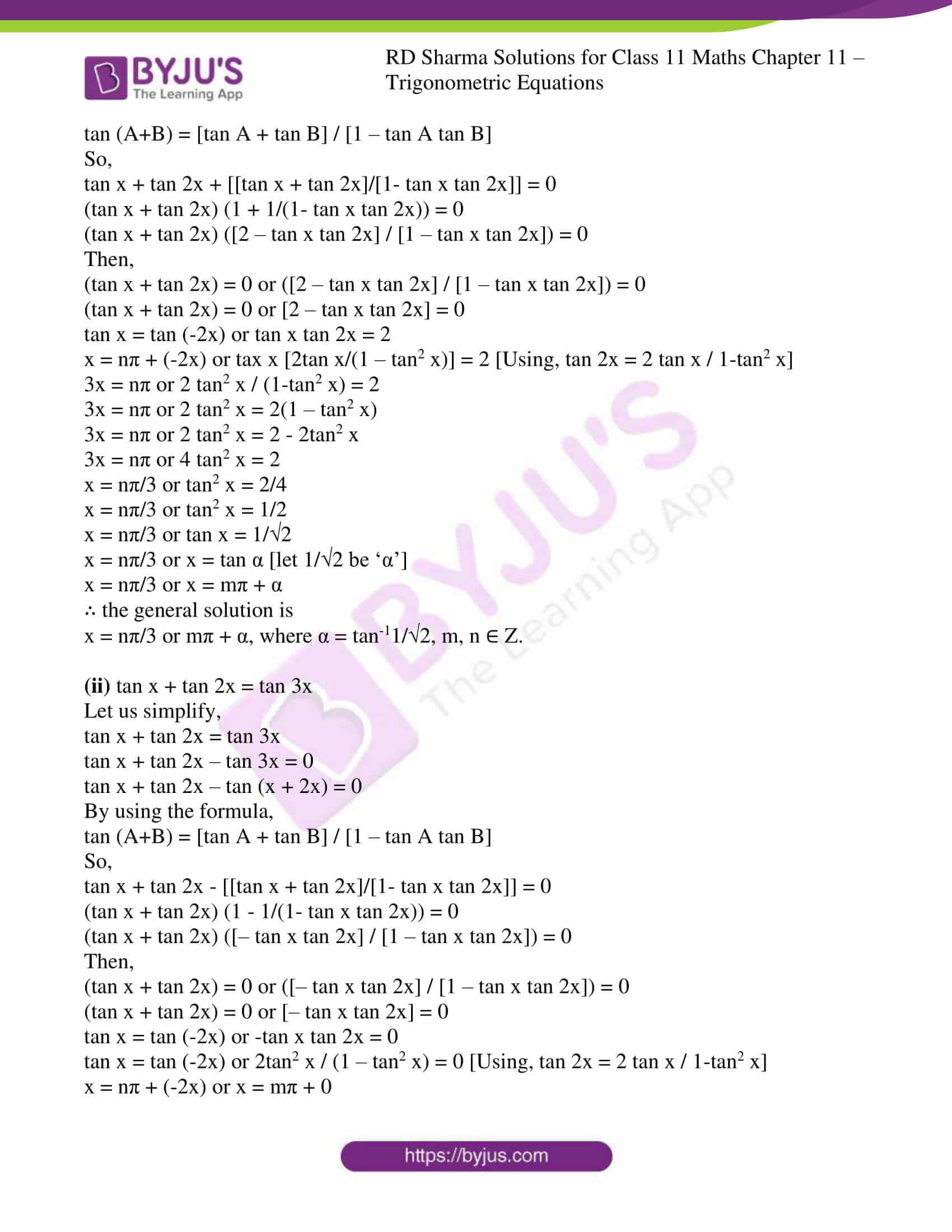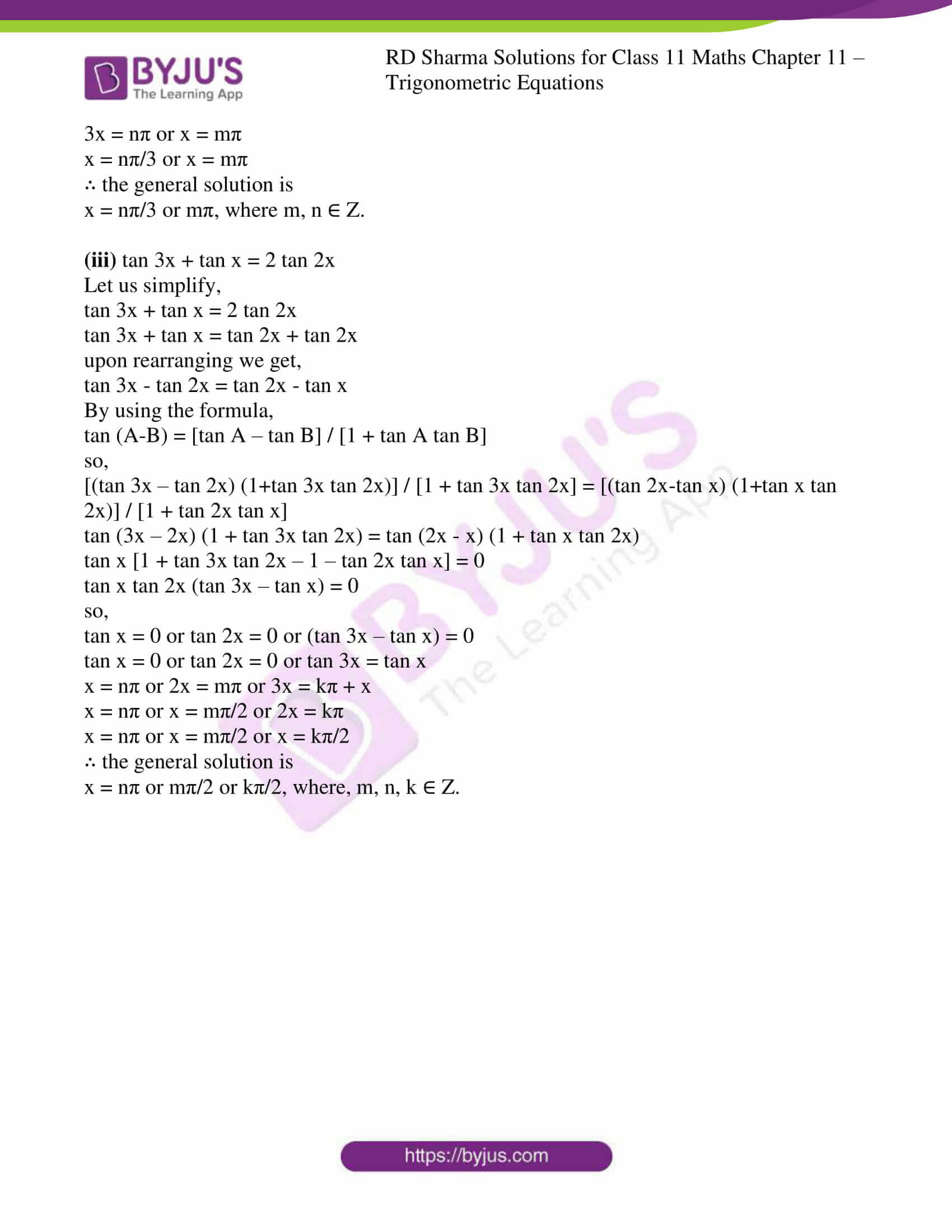### Access answers to RD Sharma Solutions for Class 11 Maths Chapter 11 – Trigonometric Equations

1. Find the general solutions of the following equations:

(i) sin x = 1/2

(ii) cos x = – √3/2

(iii) cosec x = – √2

(iv) sec x = √2

(v) tan x = -1/√3

(vi) √3 sec x = 2

Solution:

The general solution of any trigonometric equation is given as:

sin x = sin y, implies x = nπ + (– 1)n y, where n ∈ Z.

cos x = cos y, implies x = 2nπ ± y, where n ∈ Z.

tan x = tan y, implies x = nπ + y, where n ∈ Z.

(i) sin x = 1/2

We know sin 30o = sin π/6 = ½

So,

Sin x = sin π/6

∴ the general solution is

x = nπ + (– 1) n π/6, where n ∈ Z. [since, sin x = sin A => x = nπ + (– 1) n A]

(ii) cos x = – √3/2

We know, cos 150o = (- √3/2) = cos 5π/6

So,

Cos x = cos 5π/6

∴ the general solution is

x = 2nπ ± 5π/6, where n ϵ Z.

(iii) cosec x = – √2

Let us simplify,

1/sin x = – √2 [since, cosec x = 1/sin x]

Sin x = -1/√2

= sin [π + π/4]

= sin 5π/4 or sin (-π/4)

∴ the general solution is

x = nπ + (-1)n+1 π/4, where n ϵ Z.

(iv) sec x = √2

Let us simplify,

1/cos x = √2 [since, sec x = 1/cos x]

Cos x = 1/√2

= cos π/4

∴ the general solution is

x = 2nπ ± π/4, where n ϵ Z.

(v) tan x = -1/√3

Let us simplify,

tan x = -1/√3

tan x = tan (π/6)

= tan (-π/6) [since, tan (-x) = -tan x]

∴ the general solution is

x = nπ + (-π/6), where n ϵ Z.

or x = nπ – π/6, where n ϵ Z.

(vi) √3 sec x = 2

Let us simplify,

sec x = 2/√3

1/cos x = 2/√3

Cos x = √3/2

= cos (π/6)

∴ the general solution is

x = 2nπ ± π/6, where n ϵ Z.

2. Find the general solutions of the following equations:

(i) sin 2x = √3/2

(ii) cos 3x = 1/2

(iii) sin 9x = sin x

(iv) sin 2x = cos 3x

(v) tan x + cot 2x = 0

(vi) tan 3x = cot x

(vii) tan 2x tan x = 1

(viii) tan mx + cot nx = 0

(ix) tan px = cot qx

(x) sin 2x + cos x = 0

(xi) sin x = tan x

(xii) sin 3x + cos 2x = 0

Solution:

The general solution of any trigonometric equation is given as:

sin x = sin y, implies x = nπ + (– 1)n y, where n ∈ Z.

cos x = cos y, implies x = 2nπ ± y, where n ∈ Z.

tan x = tan y, implies x = nπ + y, where n ∈ Z.

(i) sin 2x = √3/2

Let us simplify,

sin 2x = √3/2

= sin (π/3)

∴ the general solution is

2x = nπ + (-1)n π/3, where n ϵ Z.

x = nπ/2 + (-1)n π/6, where n ϵ Z.

(ii) cos 3x = 1/2

Let us simplify,

cos 3x = 1/2

= cos (π/3)

∴ the general solution is

3x = 2nπ ± π/3, where n ϵ Z.

x = 2nπ/3 ± π/9, where n ϵ Z.

(iii) sin 9x = sin x

Let us simplify,

Sin 9x – sin x = 0

Using transformation formula,

Sin A – sin B = 2 cos (A+B)/2 sin (A-B)/2

So,

= 2 cos (9x+x)/2 sin (9x-x)/2

=> cos 5x sin 4x = 0

Cos 5x = 0 or sin 4x = 0

Let us verify both the expressions,

Cos 5x = 0

Cos 5x = cos π/2

5x = (2n + 1)π/2

x = (2n + 1)π/10, where n ϵ Z.

sin 4x = 0

sin 4x = sin 0

4x = nπ

x = nπ/4, where n ϵ Z.

∴ the general solution is

x = (2n + 1)π/10 or nπ/4, where n ϵ Z.

(iv) sin 2x = cos 3x

Let us simplify,

sin 2x = cos 3x

cos (π/2 – 2x) = cos 3x [since, sin A = cos (π/2 – A)]

π/2 – 2x = 2nπ ± 3x

π/2 – 2x = 2nπ + 3x [or] π/2 – 2x = 2nπ – 3x

5x = π/2 + 2nπ [or] x = 2nπ – π/2

5x = π/2 (1 + 4n) [or] x = π/2 (4n – 1)

x = π/10 (1 + 4n) [or] x = π/2 (4n – 1)

∴ the general solution is

x = π/10 (4n + 1) [or] x = π/2 (4n – 1), where n ϵ Z.

(v) tan x + cot 2x = 0

Let us simplify,

tan x = – cot 2x

tan x = – tan (π/2 – 2x) [since, cot A = tan (π/2 – A)]

tan x = tan (2x – π/2) [since, – tan A = tan -A]

x = nπ + 2x – π/2

x = nπ – π/2

∴ the general solution is

x = nπ – π/2, where n ϵ Z.

(vi) tan 3x = cot x

Let us simplify,

tan 3x = cot x

tan 3x = tan (π/2 – x) [since, cot A = tan (π/2 – A)]

3x = nπ + π/2 – x

4x = nπ + π/2

x = nπ/4 + π/8

∴ the general solution is

x = nπ/4 + π/8, where n ϵ Z.

(vii) tan 2x tan x = 1

Let us simplify,

tan 2x tan x = 1

tan 2x = 1/tan x

= cot x

tan 2x = tan (π/2 – x) [since, cot A = tan (π/2 – A)]

2x = nπ + π/2 – x

3x = nπ + π/2

x = nπ/3 + π/6

∴ the general solution is

x = nπ/3 + π/6, where n ϵ Z.

(viii) tan mx + cot nx = 0

Let us simplify,

tan mx + cot nx = 0

tan mx = – cot nx

= – tan (π/2 – nx) [since, cot A = tan (π/2 – A)]

tan mx = tan (nx + π/2) [since, – tan A = tan -A]

mx = kπ + nx + π/2

(m – n) x = kπ + π/2

(m – n) x = π (2k + 1)/2

x = π (2k + 1)/2(m – n)

∴ the general solution is

x = π (2k + 1)/2(m – n), where m, n, k ϵ Z.

(ix) tan px = cot qx

Let us simplify,

tan px = cot qx

tan px = tan (π/2 – qx) [since, cot A = tan (π/2 – A)]

px = nπ ± (π/2 – qx)

(p + q) x = nπ + π/2

x = nπ/(p+q) + π/2(p+q)

= π (2n +1)/ 2(p+q)

∴ the general solution is

x = π (2n +1)/ 2(p+q), where n ϵ Z.

(x) sin 2x + cos x = 0

Let us simplify,

sin 2x + cos x = 0

cos x = – sin 2x

cos x = – cos (π/2 – 2x) [since, sin A = cos (π/2 – A)]

= cos (π – (π/2 – 2x)) [since, -cos A = cos (π – A)]

= cos (π/2 + 2x)

x = 2nπ ± (π/2 + 2x)

So,

x = 2nπ + (π/2 + 2x) [or] x = 2nπ – (π/2 + 2x)

x = – π/2 – 2nπ [or] 3x = 2nπ – π/2

x = – π/2 (1 + 4n) [or] x = π/6 (4n – 1)

∴ the general solution is

x = – π/2 (1 + 4n), where n ϵ Z. [or] x = π/6 (4n – 1)

x = π/2 (4n – 1), where n ϵ Z. [or] x = π/6 (4n – 1), where n ϵ Z.

(xi) sin x = tan x

Let us simplify,

sin x = tan x

sin x = sin x/cos x

sin x cos x = sin x

sin x (cos x – 1) = 0

So,

Sin x = 0 or cos x – 1 = 0

Sin x = sin 0 [or] cos x = 1

Sin x = sin 0 [or] cos x = cos 0

x = nπ [or] x = 2mπ

∴ the general solution is

x = nπ [or] 2mπ, where n, m ϵ Z.

(xii) sin 3x + cos 2x = 0

Let us simplify,

sin 3x + cos 2x = 0

cos 2x = – sin 3x

cos 2x = – cos (π/2 – 3x) [since, sin A = cos (π/2 – A)]

cos 2x = cos (π – (π/2 – 3x)) [since, -cos A = cos (π – A)]

cos 2x = cos (π/2 + 3x)

2x = 2nπ ± (π/2 + 3x)

So,

2x = 2nπ + (π/2 + 3x) [or] 2x = 2nπ – (π/2 + 3x)

x = -π/2 – 2nπ [or] 5x = 2nπ – π/2

x = -π/2 (1 + 4n) [or] x = π/10 (4n – 1)

x = – π/2 (4n + 1) [or] π/10 (4n – 1)

∴ the general solution is

x = – π/2 (4n + 1) [or] π/10 (4n – 1)

x = π/2 (4n – 1) [or] π/10 (4n – 1), where n ϵ Z.

3. Solve the following equations:

(i) sin2 x – cos x = 1/4

(ii) 2 cos2 x – 5 cos x + 2 = 0

(iii) 2 sin2 x + √3 cos x + 1 = 0

(iv) 4 sin2 x – 8 cos x + 1 = 0

(v) tan2 x + (1 – √3) tan x – √3 = 0

(vi) 3 cos2 x – 2√3 sin x cos x – 3 sin2 x = 0

(vii) cos 4x = cos 2x

Solution:

The general solution of any trigonometric equation is given as:

sin x = sin y, implies x = nπ + (– 1)n y, where n ∈ Z.

cos x = cos y, implies x = 2nπ ± y, where n ∈ Z.

tan x = tan y, implies x = nπ + y, where n ∈ Z.

(i) sin2 x – cos x = 1/4

Let us simplify,

sin2 x – cos x = ¼

1 – cos2 x – cos x = 1/4 [since, sin2 x = 1 – cos2 x]

4 – 4 cos2 x – 4 cos x = 1

4cos2 x + 4cos x – 3 = 0

Let cos x be ‘k’

So,

4k2 + 4k – 3 = 0

4k2 – 2k + 6k – 3 = 0

2k (2k – 1) + 3 (2k – 1) = 0

(2k – 1) + (2k + 3) = 0

(2k – 1) = 0 or (2k + 3) = 0

k = 1/2 or k = -3/2

cos x = 1/2 or cos x = -3/2

we shall consider only cos x = 1/2. cos x = -3/2 is not possible.

so,

cos x = cos 60o = cos π/3

x = 2nπ ± π/3

∴ the general solution is

x = 2nπ ± π/3, where n ϵ Z.

(ii) 2 cos2 x – 5 cos x + 2 = 0

Let us simplify,

2 cos2 x – 5 cos x + 2 = 0

Let cos x be ‘k’

2k2 – 5k + 2 = 0

2k2 – 4k – k +2 = 0

2k(k – 2) -1(k -2) = 0

(k – 2) (2k – 1) = 0

k = 2 or k = 1/2

cos x = 2 or cos x = 1/2

we shall consider only cos x = 1/2. cos x = 2 is not possible.

so,

cos x = cos 60o = cos π/3

x = 2nπ ± π/3

∴ the general solution is

x = 2nπ ± π/3, where n ϵ Z.

(iii) 2 sin2 x + √3 cos x + 1 = 0

Let us simplify,

2 sin2 x + √3 cos x + 1 = 0

2 (1 – cos2 x) + √3 cos x + 1 = 0 [since, sin2 x = 1 – cos2 x]

2 – 2 cos2 x + √3 cos x + 1 = 0

2 cos2 x – √3 cos x – 3 = 0

Let cos x be ‘k’

2k2 – √3 k – 3 = 0

2k2 -2√3 k + √3 k – 3 = 0

2k(k – √3) +√3(k – √3) = 0

(2k + √3) (k – √3) = 0

k = √3 or k = -√3/2

cos x = √3 or cos x = -√3/2

we shall consider only cos x = -√3/2. cos x = √3 is not possible.

so,

cos x = -√3/2

cos x = cos 150° = cos 5π/6

x = 2nπ ± 5π/6, where n ϵ Z.

(iv) 4 sin2 x – 8 cos x + 1 = 0

Let us simplify,

4 sin2 x – 8 cos x + 1 = 0

4 (1 – cos2 x) – 8 cos x + 1 = 0 [since, sin2 x = 1 – cos2 x]

4 – 4 cos2 x – 8 cos x + 1 = 0

4 cos2 x + 8 cos x – 5 = 0

Let cos x be ‘k’

4k2 + 8k – 5 = 0

4k2 -2k + 10k – 5 = 0

2k(2k – 1) + 5(2k – 1) = 0

(2k + 5) (2k – 1) = 0

k = -5/2 = -2.5 or k = 1/2

cos x = -2.5 or cos x = 1/2

we shall consider only cos x = 1/2. cos x = -2.5 is not possible.

so,

cos x = cos 60o = cos π/3

x = 2nπ ± π/3

∴ the general solution is

x = 2nπ ± π/3, where n ϵ Z.

(v) tan2 x + (1 – √3) tan x – √3 = 0

Let us simplify,

tan2 x + (1 – √3) tan x – √3 = 0

tan2 x + tan x – √3 tan x – √3 = 0

tan x (tan x + 1) – √3 (tan x + 1) = 0

(tan x + 1) ( tan x – √3) = 0

tan x = -1 or tan x = √3

As, tan x ϵ (-∞ , ∞) so both values are valid and acceptable.

tan x = tan (-π/4) or tan x = tan (π/3)

x = mπ – π/4 or x = nπ + π/3

∴ the general solution is

x = mπ – π/4 or nπ + π/3, where m, n ϵ Z.

(vi) 3 cos2 x – 2√3 sin x cos x – 3 sin2 x = 0

Let us simplify,

3 cos2 x – 2√3 sin x cos x – 3 sin2 x = 0

3 cos2 x – 3√3 sin x cos x + √3 sin x cos x – 3 sin2 x = 0

3 cos x (cos x – √3sin x) + √3 sin x (cos x – √3 sin x) = 0

√3 (cos x – √3 sin x) (√3 cos x + sin x) = 0

cos x – √3 sin x = 0 or sin x + √3 cos x = 0

cos x = √3 sin x or sin x = -√3 cos x

tan x = 1/√3 or tan x = -√3

As, tan x ϵ (-∞ , ∞) so both values are valid and acceptable.

tan x = tan (π/6) or tan x = tan (-π/3)

x = mπ + π/6 or x = nπ – π/3

∴ the general solution is

x = mπ + π/6 or nπ – π/3, where m, n ϵ Z.

(vii) cos 4x = cos 2x

Let us simplify,

cos 4x = cos 2x

4x = 2nπ ± 2x

So,

4x = 2nπ + 2x [or] 4x = 2nπ – 2x

2x = 2nπ [or] 6x = 2nπ

x = nπ [or] x = nπ/3

∴ the general solution is

x = nπ [or] nπ/3, where n ϵ Z.

4. Solve the following equations:

(i) cos x + cos 2x + cos 3x = 0

(ii) cos x + cos 3x – cos 2x = 0

(iii) sin x + sin 5x = sin 3x

(iv) cos x cos 2x cos 3x = 1/4

(v) cos x + sin x = cos 2x + sin 2x

(vi) sin x + sin 2x + sin 3x = 0

(vii) sin x + sin 2x + sin 3x + sin 4x = 0

(viii) sin 3x – sin x = 4 cos2 x – 2

(ix) sin 2x – sin 4x + sin 6x = 0

Solution:

The general solution of any trigonometric equation is given as:

sin x = sin y, implies x = nπ + (– 1)n y, where n ∈ Z.

cos x = cos y, implies x = 2nπ ± y, where n ∈ Z.

tan x = tan y, implies x = nπ + y, where n ∈ Z.

(i) cos x + cos 2x + cos 3x = 0

Let us simplify,

cos x + cos 2x + cos 3x = 0

we shall rearrange and use transformation formula

cos 2x + (cos x + cos 3x) = 0

by using the formula, cos A + cos B = 2 cos (A+B)/2 cos (A-B)/2

cos 2x + 2 cos (3x+x)/2 cos (3x-x)/2 = 0

cos 2x + 2cos 2x cos x = 0

cos 2x ( 1 + 2 cos x) = 0

cos 2x = 0 or 1 + 2cos x = 0

cos 2x = cos 0 or cos x = -1/2

cos 2x = cos π/2 or cos x = cos (π – π/3)

cos 2x = cos π/2 or cos x = cos (2π/3)

2x = (2n + 1) π/2 or x = 2mπ ± 2π/3

x = (2n + 1) π/4 or x = 2mπ ± 2π/3

∴ the general solution is

x = (2n + 1) π/4 or 2mπ ± 2π/3, where m, n ϵ Z.

(ii) cos x + cos 3x – cos 2x = 0

Let us simplify,

cos x + cos 3x – cos 2x = 0

we shall rearrange and use transformation formula

cos x – cos 2x + cos 3x = 0

– cos 2x + (cos x + cos 3x) = 0

By using the formula, cos A + cos B = 2 cos (A+B)/2 cos (A-B)/2

– cos 2x + 2 cos (3x+x)/2 cos (3x-x)/2 = 0

– cos 2x + 2cos 2x cos x = 0

cos 2x ( -1 + 2 cos x) = 0

cos 2x = 0 or -1 + 2cos x = 0

cos 2x = cos 0 or cos x = 1/2

cos 2x = cos π/2 or cos x = cos (π/3)

2x = (2n + 1) π/2 or x = 2mπ ± π/3

x = (2n + 1) π/4 or x = 2mπ ± π/3

∴ the general solution is

x = (2n + 1) π/4 or 2mπ ± π/3, where m, n ϵ Z.

(iii) sin x + sin 5x = sin 3x

Let us simplify,

sin x + sin 5x = sin 3x

sin x + sin 5x – sin 3x = 0

we shall rearrange and use transformation formula

– sin 3x + sin x + sin 5x = 0

– sin 3x + (sin 5x + sin x) = 0

By using the formula, sin A + sin B = 2 sin (A+B)/2 cos (A-B)/2

– sin 3x + 2 sin (5x+x)/2 cos (5x-x)/2 = 0

2sin 3x cos 2x – sin 3x = 0

sin 3x ( 2cos 2x – 1) = 0

sin 3x = 0 or 2cos 2x – 1 = 0

sin 3x = sin 0 or cos 2x = 1/2

sin 3x = sin 0 or cos 2x = cos π/3

3x = nπ or 2x = 2mπ ± π/3

x = nπ/3 or x = mπ ± π/6

∴ the general solution is

x = nπ/3 or mπ ± π/6, where m, n ϵ Z.

(iv) cos x cos 2x cos 3x = 1/4

Let us simplify,

cos x cos 2x cos 3x = 1/4

4 cos x cos 2x cos 3x – 1 = 0

By using the formula,

2 cos A cos B = cos (A + B) + cos (A – B)

2(2cos x cos 3x) cos 2x – 1 = 0

2(cos 4x + cos 2x) cos2x – 1 = 0

2(2cos2 2x – 1 + cos 2x) cos 2x – 1 = 0 [using cos 2A = 2cos2A – 1]

4cos3 2x – 2cos 2x + 2cos2 2x – 1 = 0

2cos2 2x (2cos 2x + 1) -1(2cos 2x + 1) = 0

(2cos2 2x – 1) (2 cos 2x + 1) = 0

So,

2cos 2x + 1 = 0 or (2cos2 2x – 1) = 0

cos 2x = -1/2 or cos 4x = 0 [using cos 2θ = 2cos2θ – 1]

cos 2x = cos (π – π/3) or cos 4x = cos π/2

cos 2x = cos 2π/3 or cos 4x = cos π/2

2x = 2mπ ± 2π/3 or 4x = (2n + 1) π/2

x = mπ ± π/3 or x = (2n + 1) π/8

∴ the general solution is

x = mπ ± π/3 or (2n + 1) π/8, where m, n ϵ Z.

(v) cos x + sin x = cos 2x + sin 2x

Let us simplify,

cos x + sin x = cos 2x + sin 2x

upon rearranging we get,

cos x – cos 2x = sin 2x – sin x

By using the formula,

sin A – sin B = 2 cos (A+B)/2 sin (A-B)/2

cos A – cos B = – 2 sin (A+B)/2 sin (A-B)/2

So,

-2 sin (2x+x)/2 sin (2x-x)/2 = 2 cos (2x+x)/2 sin (2x-x)/2

2 sin 3x/2 sin x/2 = 2 cos 3x/2 sin x/2

Sin x/2 (sin 3x/2 – cos 3x/2) = 0

So,

Sin x/2 = 0 or sin 3x/2 = cos 3x/2

Sin x/2 = sin mπ or sin 3x/2 / cos 3x/2 = 0

Sin x/2 = sin mπ or tan 3x/2 = 1

Sin x/2 = sin mπ or tan 3x/2 = tan π/4

x/2 = mπ or 3x/2 = nπ + π/4

x = 2mπ or x = 2nπ/3 + π/6

∴ the general solution is

x = 2mπ or 2nπ/3 + π/6, where m, n ϵ Z.

(vi) sin x + sin 2x + sin 3x = 0

Let us simplify,

sin x + sin 2x + sin 3x = 0

we shall rearrange and use transformation formula

sin 2x + sin x + sin 3x = 0

By using the formula,

sin A + sin B = 2 sin (A+B)/2 cos (A-B)/2

So,

Sin 2x + 2 sin (3x+x)/2 cos (3x-x)/2 = 0

Sin 2x + 2sin 2x cos x = 0

Sin 2x (2 cos x + 1) = 0

Sin 2x = 0 or 2cos x + 1 = 0

Sin 2x = sin 0 or cos x = -1/2

Sin 2x = sin 0 or cos x = cos (π – π/3)

Sin 2x = sin 0 or cos x = cos 2π/3

2x = nπ or x = 2mπ ± 2π/3

x = nπ/2 or x = 2mπ ± 2π/3

∴ the general solution is

x = nπ/2 or 2mπ ± 2π/3, where m, n ϵ Z.

(vii) sin x + sin 2x + sin 3x + sin 4x = 0

Let us simplify,

sin x + sin 2x + sin 3x + sin 4x = 0

we shall rearrange and use transformation formula

sin x + sin 3x + sin 2x + sin 4x = 0

By using the formula,

sin A + sin B = 2 sin (A+B)/2 cos (A-B)/2

So,

2 sin (3x+x)/2 cos (3x-x)/2 + 2 sin (4x+2x)/2 cos (4x-2x)/2 = 0

2 sin 2x cos x + 2 sin 3x cos x = 0

2cos x (sin 2x + sin 3x) = 0

Again by using the formula,

sin A + sin B = 2 sin (A+B)/2 cos (A-B)/2

we get,

2cos x (2 sin (3x+2x)/2 cos (3x-2x)/2) = 0

2cos x (2 sin 5x/2 cos x/2) = 0

4 cos x sin 5x/2 cos x/2 = 0

So,

Cos x = 0 or sin 5x/2 = 0 or cos x/2 = 0

Cos x = cos 0 or sin 5x/2 = sin 0 or cos x/2 = cos 0

Cos x = cos π/2 or sin 5x/2 = kπ or cos x/2 = cos (2p + 1) π/2

x = (2n + 1) π/2 or 5x/2 = kπ or x/2 = (2p + 1) π/2

x = (2n + 1) π/2 or x = 2kπ/5 or x = (2p + 1)

x = nπ + π/2 or x = 2kπ/5 or x = (2p + 1)

∴ the general solution is

x = nπ + π/2 or x = 2kπ/5 or x = (2p + 1), where n, k, p ϵ Z.

(viii) sin 3x – sin x = 4 cos2 x – 2

Let us simplify,

sin 3x – sin x = 4 cos2 x – 2

sin 3x – sin x = 2(2 cos2 x – 1)

sin 3x – sin x = 2 cos 2x [since, cos 2A = 2cos2 A – 1]

By using the formula,

Sin A – sin B = 2 cos (A+B)/2 sin (A-B)/2

So,

2 cos (3x+x)/2 sin (3x-x)/2 = 2 cos 2x

2 cos 2x sin x – 2 cos 2x = 0

2 cos 2x (sin x – 1) = 0

Then,

2 cos 2x = 0 or sin x – 1 = 0

Cos 2x = 0 or sin x = 1

Cos 2x = cos 0 or sin x = sin 1

Cos 2x = cos 0 or sin x = sin π/2

2x = (2n + 1) π/2 or x = mπ + (-1) m π/2

x = (2n + 1) π/4 or x = mπ + (-1) m π/2

∴ the general solution is

x = (2n + 1) π/4 or mπ + (-1) m π/2, where m, n ϵ Z.

(ix) sin 2x – sin 4x + sin 6x = 0

Let us simplify,

sin 2x – sin 4x + sin 6x = 0

we shall rearrange and use transformation formula

– sin 4x + sin 6x + sin 2x = 0

By using the formula,

sin A + sin B = 2 sin (A+B)/2 cos (A-B)/2

we get,

– sin 4x + 2 sin (6x+2x)/2 cos (6x-2x)/2 = 0

– sin 4x + 2 sin 4x cos 2x = 0

Sin 4x (2 cos 2x – 1) = 0

So,

Sin 4x = 0 or 2 cos 2x – 1 = 0

Sin 4x = sin 0 or cos 2x = 1/2

Sin 4x = sin 0 or cos 2x = π/3

4x = nπ or 2x = 2mπ ± π/3

x = nπ/4 or x = mπ ± π/6

∴ the general solution is

x = nπ/4 or mπ ± π/6, where m, n ϵ Z.

5. Solve the following equations:

(i) tan x + tan 2x + tan 3x = 0

(ii) tan x + tan 2x = tan 3x

(iii) tan 3x + tan x = 2 tan 2x

Solution:

The general solution of any trigonometric equation is given as:

sin x = sin y, implies x = nπ + (– 1)n y, where n ∈ Z.

cos x = cos y, implies x = 2nπ ± y, where n ∈ Z.

tan x = tan y, implies x = nπ + y, where n ∈ Z.

(i) tan x + tan 2x + tan 3x = 0

Let us simplify,

tan x + tan 2x + tan 3x = 0

tan x + tan 2x + tan (x + 2x) = 0

By using the formula,

tan (A+B) = [tan A + tan B] / [1 – tan A tan B]

So,

tan x + tan 2x + [[tan x + tan 2x]/[1- tan x tan 2x]] = 0

(tan x + tan 2x) (1 + 1/(1- tan x tan 2x)) = 0

(tan x + tan 2x) ([2 – tan x tan 2x] / [1 – tan x tan 2x]) = 0

Then,

(tan x + tan 2x) = 0 or ([2 – tan x tan 2x] / [1 – tan x tan 2x]) = 0

(tan x + tan 2x) = 0 or [2 – tan x tan 2x] = 0

tan x = tan (-2x) or tan x tan 2x = 2

x = nπ + (-2x) or tax x [2tan x/(1 – tan2 x)] = 2 [Using, tan 2x = 2 tan x / 1-tan2 x]

3x = nπ or 2 tan2 x / (1-tan2 x) = 2

3x = nπ or 2 tan2 x = 2(1 – tan2 x)

3x = nπ or 2 tan2 x = 2 – 2tan2 x

3x = nπ or 4 tan2 x = 2

x = nπ/3 or tan2 x = 2/4

x = nπ/3 or tan2 x = 1/2

x = nπ/3 or tan x = 1/2

x = nπ/3 or x = tan α [let 1/2 be ‘α’]

x = nπ/3 or x = mπ + α

∴ the general solution is

x = nπ/3 or mπ + α, where α = tan-11/2, m, n ∈ Z.

(ii) tan x + tan 2x = tan 3x

Let us simplify,

tan x + tan 2x = tan 3x

tan x + tan 2x – tan 3x = 0

tan x + tan 2x – tan (x + 2x) = 0

By using the formula,

tan (A+B) = [tan A + tan B] / [1 – tan A tan B]

So,

tan x + tan 2x – [[tan x + tan 2x]/[1- tan x tan 2x]] = 0

(tan x + tan 2x) (1 – 1/(1- tan x tan 2x)) = 0

(tan x + tan 2x) ([– tan x tan 2x] / [1 – tan x tan 2x]) = 0

Then,

(tan x + tan 2x) = 0 or ([– tan x tan 2x] / [1 – tan x tan 2x]) = 0

(tan x + tan 2x) = 0 or [– tan x tan 2x] = 0

tan x = tan (-2x) or -tan x tan 2x = 0

tan x = tan (-2x) or 2tan2 x / (1 – tan2 x) = 0 [Using, tan 2x = 2 tan x / 1-tan2 x]

x = nπ + (-2x) or x = mπ + 0

3x = nπ or x = mπ

x = nπ/3 or x = mπ

∴ the general solution is

x = nπ/3 or mπ, where m, n ∈ Z.

(iii) tan 3x + tan x = 2 tan 2x

Let us simplify,

tan 3x + tan x = 2 tan 2x

tan 3x + tan x = tan 2x + tan 2x

upon rearranging we get,

tan 3x – tan 2x = tan 2x – tan x

By using the formula,

tan (A-B) = [tan A – tan B] / [1 + tan A tan B]

so,

[(tan 3x – tan 2x) (1+tan 3x tan 2x)] / [1 + tan 3x tan 2x] = [(tan 2x-tan x) (1+tan x tan 2x)] / [1 + tan 2x tan x]

tan (3x – 2x) (1 + tan 3x tan 2x) = tan (2x – x) (1 + tan x tan 2x)

tan x [1 + tan 3x tan 2x – 1 – tan 2x tan x] = 0

tan x tan 2x (tan 3x – tan x) = 0

so,

tan x = 0 or tan 2x = 0 or (tan 3x – tan x) = 0

tan x = 0 or tan 2x = 0 or tan 3x = tan x

x = nπ or 2x = mπ or 3x = kπ + x

x = nπ or x = mπ/2 or 2x = kπ

x = nπ or x = mπ/2 or x = kπ/2

∴ the general solution is

x = nπ or mπ/2 or kπ/2, where, m, n, k ∈ Z.

## Frequently Asked Questions on RD Sharma Solutions for Class 11 Maths Chapter 11

### What are the key points of using the RD Sharma Solutions for Class 11 Maths Chapter 11?

The key points of using the RD Sharma Solutions for Class 11 Maths Chapter 11 are:
1. BYJU’S RD Sharma Solutions are the most reliable study guides that students can confidently rely on for exam preparations.
2. Individual subject matter experts have curated the solutions as per the CBSE guidelines and marking schemes.
3. Improves conceptual learning and analytical skills among students.
4. It provides solutions for all the textbook questions.

### Can the RD Sharma Solutions for Class 11 Maths Chapter 11 be considered as the best study material for exam preparations?

We at BYJU’S have provided solutions to all the textbook questions based on the latest CBSE guidelines. All the solutions are framed by individual subject matter experts to help students ace the exam. The answers are compiled in PDFs so that students can download them for free and refer to it anywhere and anytime. The solutions contain detailed explanations in a step by step manner to boost the confidence of students in answering the difficult and long-answer type questions efficiently.

### How can students prepare for the annual exam using the RD Sharma Solutions for Class 11 Maths Chapter 11?

Before the annual exam, students have to learn the chapter thoroughly and revise them regularly to attain a strong grip over the key concepts. The solutions provided by expert tutors at BYJU’S are an apt resource for exam preparations and to score high marks in the exam. Also, students must read thoroughly the syllabus for the academic year before the exam to understand the marks weightage for the chapter. It’s recommended that students refer to the solutions while attempting the questions of a new chapter to comprehend the method of answering as per the latest CBSE guidelines.